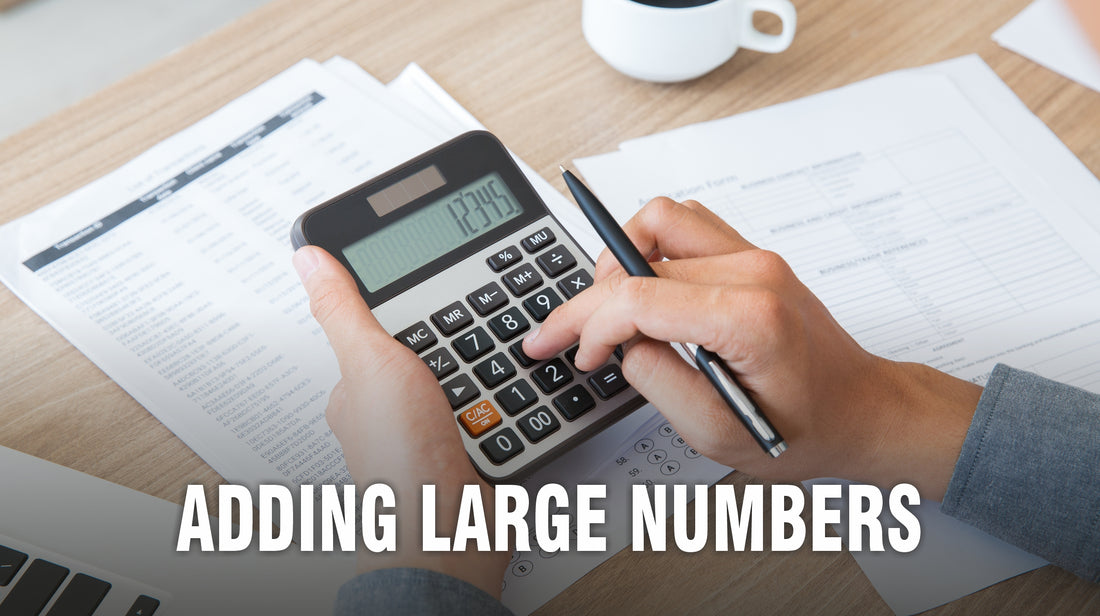Ability to add large numbers quickly in your head is a neat trick – helpful when you are grocery shopping or trying to figure out how many runs your favorite player has made in a series.

It is easier to handle numbers when they are rounded off.

So 397 becomes 400 and 918 becomes 920.

It is now easier to add 400 to 920. The total is 1320.

To arrive at the original equation, we have to figure out how much we added to our original numbers.

400 – 397 = 3 and 920 – 918 = 2. So we have added a total of 5 to our original numbers.
So we subtract  5 from 1320.

1320 – 5 = 1315.

So 397 + 918 = 1315.

Get lots of Math practice problems for standardized tests like the SAT, ACT, GRE and GMAT in the Test Prep Series books of Vibrant Publishers.